# Effects of Variable Viscosity and Thermal Conductivity on the MHD Flow of Micropolar Fluid Past an Accelerated Infinite Vertical Insulated Plate

Effects of Variable Viscosity and Thermal Conductivity on the MHD Flow of Micropolar Fluid Past an Accelerated Infinite Vertical Insulated Plate

Parash Moni Thakur G. C. Hazarika

Department of Mathematics, Dibrugarh University, Dibrugarh

Department of Mathematics, Dibrugarh University, Dibrugarh, Assam, India

Corresponding Author Email:
gchazarika@dibru.ac.in
Page:
73-78
|
DOI:
http://doi.org/10.18280/ijht.330310
N/A
|
Accepted:
N/A
|
Published:
30 September 2015
| Citation

OPEN ACCESS

Abstract:

The effects of temperature dependent viscosity and thermal conductivity on magneto hydrodynamic flow, heat and mass transfer of an incompressible micropolar fluid past an accelerated infinite vertical plate are studied where the viscosity and thermal conductivity are assumed to be inverse linear functions of temperature. The partial differential equations governing the flow, heat and mass transfer of the problem are transformed into dimensionless form of ordinary differential equations by using similarity substitutions. The governing boundary value problems are then solved numerically using shooting method. The effects of various parameters viz. viscosity parameter, thermal conductivity parameter, mass transfer parameter, coupling constant parameter, Prandtl number, Schmidt number, Reynolds number and magnetic parameter on velocity, micro-rotation, temperature and concentration fields are obtained and presented graphically. The Skin-friction, Nusselt number  and  Sherwood number are also computed and presented in tabular form.

Keywords:

Micropolar fluid, Variable viscosity and thermal conductivity, Mass transfer, MHD Flow.

1. Introduction

The theory of micropolar fluid has been a field of very active research for the last few decades. This theory, first introduced and formulated by Eringen  (1966),  is capable to explain the complex fluids behaviour such as liquid crystals, polymeric suspensions, animal blood etc. by taking into account the effect arising from local structure and micro-motions of the fluid elements. In the theory of micropolar fluid, each particle has a finite size and constitutes a micro structure, which can rotate. This local rotation of the particles is in addition to the usual rigid body motion of the entire volume element. The effect of magneto hydrodynamics on micropolar flow has become important due to several engineering applications such as in MHD generators, designing cooling system for nuclear reactor, flow meters etc., where the micro concentration provides an important parameter  for deciding the rate of heat flow. Several investigators have made theoretical and experimental studies of micropolar flow in the presence of a transverse magnetic field during the last decades. Assuming fluid viscosity as a linear function of temperature the effect of variable viscosity on MHD natural convection in micropolar fluids was studied by Abd El-Hakiem et al. . Ahmed and Kalita  studied MHD oscillatory free convective flow past a vertical plate in slip- flow regime with variable suction and periodic plate temperature. MHD free and forced convection and mass transfer flow past a porous vertical plate was investigated by Ahmed and Hazarika . Gorla et al.  investigated the magneto hydrodynamic free convection boundary layer flow of a thermomicropolar fluid over a vertical plate. Muthucumaraswami et al.  investigated the unsteady flow past an accelerated infinite vertical plate with variable temperature and uniform mass diffusion. Rajesh  investigated the MHD free convection flow past an accelerated vertical porous plate with variable temperature through a porous medium. Using similarity substitutions and applying shooting method Sarma and Hazarika  investigated effects of variable viscosity and thermal conductivity on the flow of Newtonian fluid past an accelerated vertical insulated plate.

2. Objective

The main objective of the present work is to extend the work of Sarma and Hazarika  for the study of the effects of variable viscosity and thermal conductivity on the MHD flow of micropolar fluid past an accelerated infinite vertical insulated plate. Viscosity and thermal conductivity are assumed to be inverse linear functions of temperature. The governing partial differential equations are reduced in to ordinary differential equations by similarity transformations. The problem is then solved numerically using Runge-Kutta shooting algorithm.

3. Mathematical Formulation of the Problem

The general equations of fluid motion for two dimensional unsteady flows in Cartesian co-ordinate are considered with $x-$ axis along the vertical plate in the upward direction and the $y-$ axis normal to it, where the fluid properties, viscosity, thermal conductivity and concentration are assumed to vary with temperature.  At time $t$ >0 the infinite plate starts moving with a velocity $u=c_{0} t$  (where $c_{0}$  is a positive constant with the dimention of acceleration). As the velocity of the fluid is low, so the viscous dissipative heat is neglected. Also a magnetic field of constant intensity is assumed to be applied normal to the vertical plate and the electrical conductivity of the fluid is assumed to be so small that the induced magnetic field can be neglected in comparison to the applied magnetic field. The applied magnetic field is primary in the $y-$ direction and is a function of $t$  only. $(u(y, t), 0)$ are the velocity components and $N$ is the component of micro-rotation perpendicular to the $x y-$ plane. Under these assumptions the governing equations of the problem are considered as follows:

3.1 Governing equations

Momentum equation:

$\frac{\partial u}{\partial t}=g_{0} \beta\left(T-T_{\infty}\right)+g_{0} \beta^{*}\left(C-C_{\infty}\right)+\frac{\partial}{\partial y}\left(v \frac{\partial u}{\partial y}\right)+\frac{\kappa}{\rho}\left(\frac{\partial N}{\partial y}+\frac{\partial^{2} u}{\partial y^{2}}\right)-\frac{\sigma B^{2}}{\rho} u$   (1)

Angular momentum equation:

$\frac{\partial N}{\partial t}=\frac{\gamma}{\rho \mathrm{j}} \frac{\partial^{2} N}{\partial y^{2}}-\frac{\kappa}{\rho \mathrm{j}}\left(2 N+\frac{\partial u}{\partial y}\right)$   (2)

Energy equation:

$\frac{\partial T}{\partial t}=\frac{1}{\rho c_{p}} \frac{\partial}{\partial y}\left(\lambda \frac{\partial T}{\partial y}\right)$   (3)

Species continuity equation:

$\frac{\partial C}{\partial t}=\frac{1}{S_{c}} \frac{\partial}{\partial y}\left(v \frac{\partial C}{\partial y}\right)$     (4)

3.2 Boundary conditions

The appropriate boundary conditions are:

$\left.\begin{array}{l}u(0, t)=0, T(0, t)=T_{w}, C(0, t)=C_{w}, N(0, t)=0 \\ u(\infty, t)=0, T(\infty, t)=0, C(\infty, t)=0, N(\infty, t)=0\end{array}\right\}$   (5)

The equation of continuity being identically satisfied by $(u(y, t), 0)$. Following Gurum  we assume that $\gamma=\left(\mu_{\infty}+\frac{\kappa}{2}\right) j=\mu_{\infty}\left(1+\frac{K_{1}}{2}\right) j$ , where $K_{1}=\frac{\kappa}{v_{\infty} \rho}$ , coupling constant parameter.

Following Lai and Kulacki  we assume that the viscosity and thermal conductivity are inverse linear functions of temperature, i.e.

$\frac{1}{\mu}=\frac{1}{\mu_{\infty}}\left[1+\delta\left(T-T_{\infty}\right)\right],$ or $\frac{1}{\mu}=a\left(T-T_{c}\right)$

where $a=\frac{\delta}{\mu_{\infty}}$ and $T_{c}=T_{\infty}-\frac{1}{\delta}$

$\frac{1}{\lambda}=\frac{1}{\lambda_{\infty}}\left[1+\xi\left(T-T_{\infty}\right)\right],$ or $\frac{1}{\lambda}=b\left(T-T_{r}\right)$

Where $b=\frac{\xi}{\lambda_{\infty}}$ and $T_{r}=T_{\infty}-\frac{1}{\xi}$

Here $a, b, T_{c}$ are constants and their values depend on the reference state and thermal properties of the fluid i.e. $v$  and $\lambda$ .In general $a, b>0$ for liquids and $a, b<0$  for gases.

We introduce the following similarity transformations:

$\eta=y \sqrt{\frac{\alpha^{2} U_{0}}{(1-\alpha t) v_{\infty} c_{0}}}$

$u=U_{0}(1-\alpha t)^{1 / 2} f(\eta)$

$N=\sqrt{\frac{U_{0}^{3} \alpha^{2}}{v_{\infty} c_{0}}} h(\eta)$

$j=\frac{v_{\infty} c_{0}}{U_{0} \alpha^{2}}(1-\alpha t) i(\eta)$

$B=\sqrt{\frac{\alpha U_{0}}{\mathrm{c}_{0}(1-\alpha t)}} B_{0}$

$C=C_{\infty}+\frac{c_{0}\left(C_{w}-C_{\infty}\right)}{\alpha U_{0}}(1-\alpha t)^{1 / 2} g(\eta)$

$T=T_{\infty}+\frac{c_{0}\left(T_{w}-T_{\infty}\right)}{\alpha U_{0}}(1-\alpha t)^{1 / 2} \theta(\eta)$

$G_{r}=\frac{g_{0} \beta\left(T_{w}-T_{\infty}\right) c_{0}^{2}(1-\alpha t)}{U_{0}^{3} \alpha^{3}}, G_{c}=\frac{g_{0} \beta^{*}\left(C_{w}-C_{\infty}\right) c_{0}^{2}(1-\alpha t)}{U_{0}^{3} \alpha^{3}}$

Introducing the above transformations in equations (1)—(4), we have the following non dimensional ordinary differential equations

$\left(1+K_{1} \frac{\theta_{c}-\theta}{\theta_{c}}\right) f^{\prime \prime}=\left[G_{r} \theta+G_{c} g+K_{1} h^{\prime}-M f-\right. \left.\frac{1}{2} K_{2} R_{e}\left(f-\eta f^{\prime}\right)\right] \frac{\theta-\theta_{c}}{\theta_{c}}+\frac{\theta^{\prime} f^{\prime}}{\theta-\theta_{c}}$     (6)

$\left(2+K_{1}\right) i h^{\prime \prime}=K_{2} R_{e}\left(\eta h^{\prime}\right) i+K_{1}\left(4 h+2 f^{\prime}\right)$   (7)

$\theta^{\prime \prime}=\frac{1}{2} K_{2} P_{e_{h}}\left(\theta-\eta \theta^{\prime}\right) \frac{\theta-\theta_{r}}{\theta_{r}}+\frac{\theta^{\prime 2}}{\theta-\theta_{r}}$    (8)

$g^{\prime \prime}=\frac{1}{2} K_{2} P_{e_{m}}\left(g-\eta g^{\prime}\right) \frac{\theta-\theta_{c}}{\theta_{c}}+\frac{g^{\prime} \theta^{\prime}}{\theta-\theta_{c}}$   (9)

The boundary conditions (5)become

$\left.\begin{array}{l}\text { As } \eta=0: f=0, g=1, \theta=1, h=0 \\ \text { As } \eta \rightarrow \infty: f=0, g=0, \theta=0, h=0\end{array}\right\}$    (10)

The physical quantities of interest in this problem are the skin–friction coefficient $c_{f}$ ,Nusselt number $N u$  and Sherwood number $S_{h}$  which indicate physically wall shear stress, rate of heat transfer and rate of mass transfer respectively.For micropolar boundary layer flow, the wall shear stress $\tau_{w}$  is given by

$\tau_{w}=\left[(\mu+k) \frac{\partial u}{\partial y}+k N\right] =\rho v_{\infty}\left(\frac{\theta_{c}}{\theta_{c}-1}+K_{1}\right) \sqrt{\frac{U_{0}^{3} \alpha^{2}}{v_{\infty} c_{0}}} f^{\prime}(0)$

The skin –friction coefficient $c_{f}$  can be defined as

$c_{f}=\frac{2 \tau_{w}}{\rho U_{o}^{2}}=2\left(\frac{\theta_{c}}{\theta_{c}-1}+K_{1}\right) R e^{-\frac{1}{2}} f^{\prime}(0)$

The heat transfer from the plate is given by

$q_{w}=-\lambda\left[\frac{\partial T}{\partial y}\right]_{y=0}=\lambda_{\infty} \frac{\theta_{r}}{1-\theta_{r}} \sqrt{\frac{U_{0} c_{0}}{v_{\infty} \alpha^{2}}} \frac{\left(T_{w}-T_{\infty}\right) \alpha}{U_{0}} \theta^{\prime}(0)$

The Nusselt number is given by

$N u=\frac{q_{w} U_{0}}{\lambda_{\infty}\left(T_{w}-T_{\infty}\right) \alpha}=\frac{\theta_{r}}{1-\theta_{r}} R e^{\frac{1}{2}} \theta^{\prime}(0)$

The mass flux at the wall is given by

$M_{w}=-D\left[\frac{\partial c}{\partial y}\right]_{y=0}=-\frac{v}{S_{c}} \sqrt{\frac{U_{0} c_{0}}{v_{\infty} \alpha^{2}}} \frac{\left(c_{w}-c_{\infty}\right) \alpha}{U_{0}} g^{\prime}(0)$

$S_{h}=\frac{s_{c} M_{w} U_{0}}{v_{\infty}\left(c_{w}-C_{\infty}\right) \alpha}=\frac{\theta_{c}}{1-\theta_{c}} \operatorname{Re}^{\frac{1}{2}} g^{\prime}(0)$

4. Results and Discussion

4.1 Discussion

The equations (6)-(9) together with the boundary conditions (10) are solved for various combinations of the parameters involved in the equations using an algorithm based on the shooting method and results are displayed in the form graph for the dimensionless velocity distribution, dimensionless micro-rotation distribution, dimensionless species concentration distribution, dimensionless temperature distribution with the variation of different parameters.

The values of different parameters have been taken as $R_{e}=0.10, G_{r}=0.10, G_{c}=0.10, M=1, \operatorname{Pr}=0.70, \mathrm{Sc}=1, K_{1}=0.10, K_{2}=0.10$ with the viscosity parameter $\theta_{c}$  ranging from -10 to -1 at certain value of $\theta_{r}=-10$  unless otherwise stated. Similarly solutions have been found with the thermal conductivity parameter $\theta_{r}$ ranging from -15.00 to -1.00 at certain value of $\theta_{c}=-10.00$  keeping the other values remaining same. Solutions have also been found for different values of magnetic parameter (M), Prandtl number($P_{r}$) , Reynolds number ($R_{e}$) ,coupling constant parameter ($K_{1}$)and Schmidt number(Sc). The variations in velocity distribution, micro-rotation distribution, species concentration distribution and temperature distribution are illustrated in figures (1)—(14) with the variation of different parameters.

The figures (1)—(6) represent the variations in dimensionless velocity distribution with the variation of dimensionless reference temperature corresponding to viscosity parameter $\theta_{c}$ , dimensionless reference temperature corresponding to thermal conductivity parameter $\theta_{r}$  Reynolds number $R_{e}$  the coupling constant parameter $K_{1}$  magnetic parameter M and Schmidt number  Sc. From figure (1) it is clear that velocity increases with the increasing values $\theta_{c}$  This is due to the fact that when the temperature increases viscosity decreases and therefore velocity increases. From figure (2) it is observed that velocity decreases with the increasing values of $\theta_{r}$. It is due to the reason that  temperature decreases with the increasing values of thermal conductivity and as a result viscosity increases and velocity decreases. Figure (3) represents the distribution of velocity with the variation of Reynolds number $R_{e}$ . For small values of $R_{e}$  viscous force is predominant to inertia force and for increasing values of $R_{e}$  viscous force will be decreasing and as a result velocity increases. From figure (4) it is found that velocity decreases with increasing value of coupling constant parameter $K_{1}$ . For increasing values of this parameter vortex viscosity increases and therefore velocity decreases. From figure (5) we have observed that velocity decreases with the increasing value of magnetic parameter M. It is due to the fact that the application of transverse magnetic field will result a resistive force (Lorentz force) similar to drag force, which tends to resist the fluid flow and thus reducing its velocity. It is also observed that the velocity is maximum near the plate and decreases away from the plate and finally takes asymptotic value. As the viscosity increases with the increasing values of Schmidt number, from figure (6) we have observed that velocity decreases with the increasing values of Schmidt number.

Figures (7)—(10) display the distributions  representing micro-rotation within the boundary layer with the variation of $\theta_{r}, \theta_{c}, K_{1}$ and $M$. From these figures we have observed that the micro-rotation near the surface increases for increasing values of the different parameters and then decreases gradually.It is to be observed that at certain point the parameters have no effect on the micro-rotation distribution. The effect of the Hartmann number $M$  on micro-rotation is shown in the figure (10). The values of micro-rotation are negative in the first half whereas in the second half these are positive, thus showing a reverse rotation nears the boundary. An increase in magnetic field leads to a decrease in micro-rotation.

Figures (11) and (12) display the variations of dimensionless temperature profile $\theta(\eta)$  with the variation of dimensionless reference temperature corresponding to thermal conductivity parameter $\theta_{r}$  and Prandtl number $P_{r}$ .  From figure (11) we have observed that temperature decreases when $\theta_{r}$  increases. It is due to the fact that the kinematic viscosity of the fluid increases with the increase of $\theta_{r}$  and as a result temperature decreases. It is observed from the figure (12) that temperature increases with the increasing values of $P_{r}$  .It is due to the reason that with the increasing values of the Prandtl number the  thermal diffusivity of the fluid decreases and as a result thermal conductivity decreases. Therefore, the volumetric heat capacity of the fluid becomes larger.

Figures (13) and (14) display the distributions representing concentration profile within the boundary layer with the variation of $S_{c}$ and $R_{e}$ . It is observed that concentration increases with the increasing values of $S_{c}$ and $R_{e}$ With the increasing value of Sc mass diffusivity decreases and as a result concentration increases.

4.2 Figures

## 1.png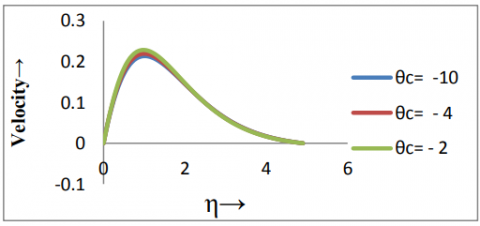Figure 1. Velocity distribution with the variation of $\theta_{c}$

## 2.pngFigure 2. Velocity distribution with the variation of $\theta_{r}$

## 3.png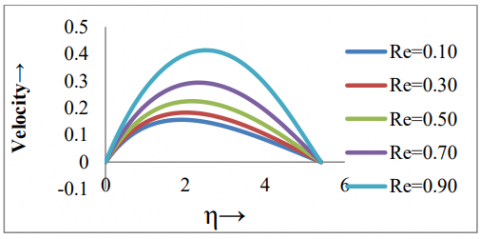Figure 3. Velocity distribution with the variation of $R_{e}$

## 4.png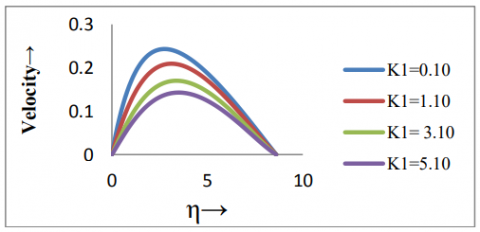Figure 4. Velocity distribution with the variation of $K_{1}$

## 5.png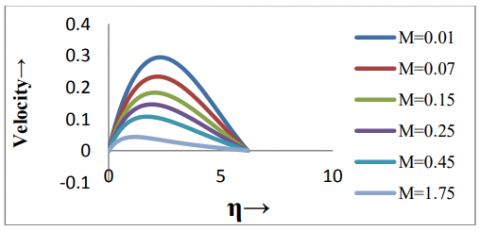Figure 5. Velocity distribution with the variation of $M$

## 6.pngFigure 6.Velocity distributions with the variation of $S_{\mathrm{C}}$

## 7.png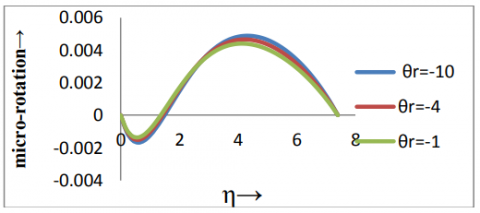Figure 7. Micro-rotation distribution with the variation of $\theta_{r}$

## 8.png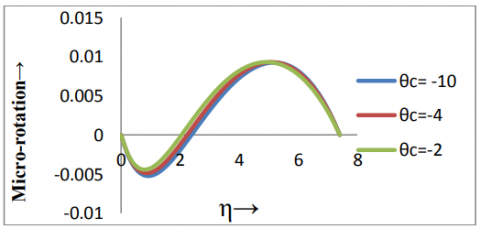Figure 8. Micro-rotation distributions with the variation of $\theta_{c}$

## 9.png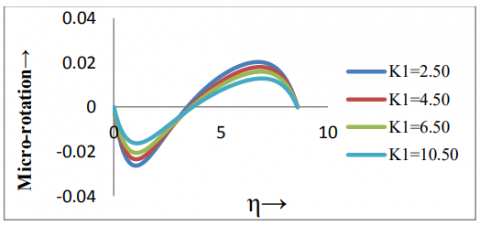Figure 9. Micro-rotation distribution with the variation of $K_{1}$

## 10.pngFigure 10. Micro-rotation distribution with  variation of $M$

## 11.pngFigure 11. Temperature distribution with the variation of $\theta_{r}$

## 12.pngFigure 12. Temperature distribution with the variation of $P_{r}$

## 13.png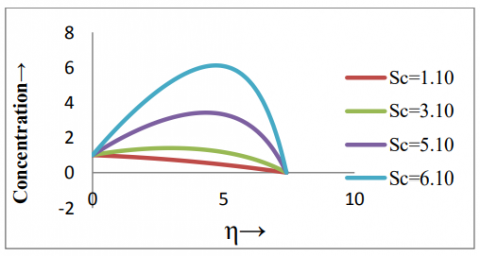Figure 13. Concentration distribution with the variation of $S c$

## 14.png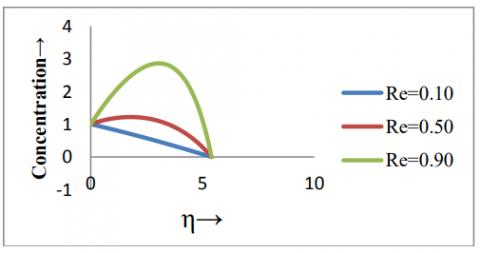Figure 14. Concentration distribution with the variation of Re

4.3 Numerical values and tables

Finally effects of the above mentioned parameters on the values of $f^{\prime}(0)$ , $g^{\prime}(0)$ , $h^{\prime}(0)$ , $\theta^{\prime}(0)$, $c_{f}$ , $N u$ and $S_{h}$  are shown in the tables (1)—(4). The behaviours of these parameters are self evident from the tables and hence any further discussions about them are seemed to be redundant.

Table 1. Variations with respect to M and $\theta_{c}$

 M $\theta_{c}$ $f^{\prime}(0)$ $g^{\prime}(0)$ $h^{\prime}(0)$ $c_{f}$ $S_{h}$ 0.1 -10 0.38813 -0.0655 -0.0289 2.47707 0.01882 2.1 -5 0.13229 -0.0854 -0.0046 0.78094 0.02251 4.1 -3 0.10135 -0.1119 -0.0025 0.54484 0.02654 6.1 -1 0.09927 -0.2177 -0.0017 0.37671 0.03442

Table 2. Variations with respect to M and $\theta_{r}$

 M $\theta_{r}$ $f^{\prime}(0)$ $g^{\prime}(0)$ $\theta^{\prime}(0)$ $c_{f}$ $N u$ $S_{h}$ 0.1 -10 0.3881 -0.0655 -0.1273 2.477 0.0365 0.0188 2.1 -5 0.1273 -0.0657 -0.1322 0.812 0.0348 0.0189 4.1 -3 0.0931 -0.0660 -0.1383 0.594 0.0328 0.0190 6.1 -1 0.0768 -0.0673 -0.1623 0.490 0.0256 0.0193

Table 3. Variations with respect to M and $P_{r}$

 Pr M $f^{\prime}(0)$ $g^{\prime}(0)$ $\theta^{\prime}(0)$ $c_{f}$ $N u$ $S_{h}$ 0.7 0.1 0.3881 -0.0655 -0.127 2.4770 0.036596 0.018829 3.7 0.3 0.2973 -0.06206 -0.059 1.897 0.01705 0.017841 7.2 0.5 0.2579 -0.05646 0.036 1.6464 -0.01058 0.016231

Table 4. Variations with respect to $P_{r}$  and $\theta_{r}$

 Pr $\theta_{r}$ $f^{\prime}(0)$ $g^{\prime}(0)$ $\theta^{\prime}(0)$ $c_{f}$ $N u$ $S_{h}$ 0.7 -10 0.2363 -0.065 -0.1273 1.5086 0.0365 0.018829 6.2 -5 0.2547 -0.058 0.0159 1.6257 -0.004 0.016714 6.7 -3 0.2587 -0.056 0.0481 1.6513 -0.011 0.016323 7.2 -1 0.2811 -0.049 0.2056 1.7943 -0.032 0.014155
5. Conclusion

In this study, the effects of variable viscosity and thermal conductivity on the flow with heat and mass transfer of an incompressible micropolar fluid past an accelerated infinite vertical insulated plate are examined. The results demonstrate clearly that the viscosity and thermal conductivity parameters along with the other parameters such as

have significant effects on velocity, temperature, concentration and micro-rotation distributions within the boundary layer. Thus presence of microconstituents may cause a significant change in flow problem.
References

1. Abd El-hakiem M., Modather M., Abdou M., The Effect of Variable Viscosity on MHD Natural Convection in Micropolarfluids, Int. J. Appl. Mech. Engg., vol.11, No. 2, pp. 301-320, 2006.

2. Ahmed N., Kalita D., MHD Oscillatory Free Convective Flow past a Vertical Plate in Slip-Flow Regime with Variable Suction and Periodic Plate Temperature, Int. J. Heat and Technology,Vol.26, No.2, pp.85-92, 2008.

3. Ahmed N., Hazarika G.C., MHD Free and Forced Convection and Mass Transfer Flow past a Porous Vertical Plate, Int. J. Heat and Technology,Vol.30, No.2, pp.97-106, 2012.

4. Eringen A.C., Theory of Micropolar Fluids, J. Math. Mech., Vol.16, pp.1-18, 1966. C.  DOI: 10.1512/iumj.1967.16.16001.

5. Gorla R. S. R., Takhar H. S., Slaouti A., Magneto Hydrodynamic Free Convection Boundary Layer Flow of a Thermo Micropolar Fluid over a Vertical Plate, Int. J. Eng. Sci., Vol.36, pp. 315-327, 1998.

6. Guram G. S. and Smith A.C., Stagnation Flow of Micropolar Fluids with Strong and Weak Interactions, Comp. Math. With Appl., Vol.6, pp. 213-233, 1980. DOI: 10.1016/0898-1221(80)90030-9.

7. Lai F. C. and Kulacki F.A., The Effect of Variable Viscosity on Convective Heat and Mass Transfer along a Vertical Surface in Saturated Porous Medium, Int. J. Heat and Mass Transfer, Vol.33, No.5, 1028-1031. DOI: 10.1016/0017-9310(90)90084-8.

8. Muthucumaraswami R., Sundar M., Subramonium V. S. A., Unsteady Flow Past an Accelerated Infinite Vertical Plate with Variable Temperature and Uniform Mass Diffusion, Int. J. Appl. Math. and Mech., Vol.5, No. 6 , pp. 51-56, 2009.

9. Rajesh V., MHD Free Convection Flow past an Accelerated Vertical Porous Plate with Variable Temperature through a Porous Medium, Acta Technica Corviniensis, Bulletin and Engineering, pp.91-96.

10. Sarma U., Hazarika G. C., Effects of Variable Viscosity and Thermal Conductivity on Flow past an Accelerated Vertical Insulated Plate, J. Rajasthan Acad. Phy. Sci., Vol.8, No.3, pp.337-348, 2009.